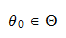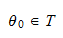StatLect

Set estimation

In statistical inference, a sample is employed to make statements about the probability distribution from which the sample has been generated (see the lecture entitled Statistical inference). The samplecan be regarded as a realization of a random vector, whose joint distribution function, denoted by, is unknown, but is assumed to belong to a set of distribution functions, called statistical model.

In a parametric model, the setis put into correspondence with a setof-dimensional real vectors.is called the parameter space and its elements are called parameters. Denote bythe true parameter, that is, the parameter that is associated with the unknown distribution functionfrom which the samplewas actually generated. For concreteness,is assumed to be unique. This lecture discusses a kind of inference aboutcalled set estimation.Confidence sets

Roughly speaking, set estimation is the act of choosing a subsetof the parameter space () in such a way thathas a high probability of containing the true (and unknown) parameter. The chosen subsetis called a set estimate ofor a confidence set for.

When the parameter spaceis a subset of the set of real numbersand the subsetis chosen among the intervals of(e.g. intervals of the kind), we speak about interval estimation (instead of set estimation), interval estimate (instead of set estimate) and confidence interval (instead of confidence set).

When the set estimateis produced using a predefined rule (a function) that associates a set estimateto eachin the support of, we can writeThe functionis called a set estimator. Often, the symbolis used to denote both the set estimate and the set estimator. The meaning is usually clear from the context.

Coverage probabilities and confidence coefficients

As we already said, set estimation is the act of choosing a subsetof the parameter space in such a way thathas a high probability of containing the true parameter. The probability thatcontains the true parameter is called coverage probability and it is usually chosen by the statistician. Intuitively, before observing the data the statistician makes a statement:whereis the parameter space, containing all the parameters that are deemed plausible. The statistician believes the statement to be true, but the statement is not very informative, becauseis a very large set. After observing the data, she makes a more informative statement:This statement is more informative, becauseis smaller than, but it has a positive probability of being wrong (which is the complement toof the coverage probability). In controlling this probability, the statistician faces a trade-off: if she decreases the probability of being wrong, then her statements become less informative; on the contrary, if she increases the probability of being wrong, then her statements become more informative.

In formal terms, the coverage probability of a set estimator is defined as follows:where the notationis used to indicate the fact that the probability is calculated using the distribution functionassociated to the true parameter. It is important to note that in the above definition of coverage probability the random quantity is the interval, while the parameter is fixed.

In practice, the coverage probability is seldom known, because it depends on the unknown parameter(although in some cases it is equal for all parameters belonging to the parameter space). When the coverage probability is not known, it is customary to compute the confidence coefficient, which is defined as follows:In other words, the confidence coefficientis equal to the smallest possible coverage probability. The confidence coefficient is also often called level of confidence.

Size of a confidence set

We already mentioned that there is a trade-off in the construction and choice of a set estimator. On the one hand, we want our set estimatorto have a high coverage probability, that is, we want the setto include the true parameter with a high probability. On the other hand, we want the size ofto be as small as possible, so as to make our interval estimate more precise. What do we mean by size of? If the parameter spaceis unidimensional andis an interval estimate, then the size ofis just its length. If the spaceis multidimensional, then the size ofis its volume. The size of a confidence set is also called measure of a confidence set (for those who have a grasp of measure theory, the name stems from the fact that Lebesgue measure is the generalization of volume in multidimensional spaces). If we denote bythe size of a confidence set, then we can also define the expected size of a set estimator:where the notationis used to indicate the fact that the expected value is calculated using the distribution functionassociated to the true parameter. Like the coverage probability, also the expected size of a set estimator depends on the unknown parameter. Hence, unless it is a constant function of, one needs to somehow estimate it or to take the infimum over all possible values of the parameter, as we did above for coverage probabilities.

Other criteria to evaluate set estimators

Although size is probably the simplest criterion to evaluate and select set estimators, there are several other criteria. We do not discuss them here, but we refer the reader to the very nice exposition in Berger, R. L. and G. Casella (2002) "Statistical inference", Duxbury Advanced Series.

Examples

Examples of set estimation problems can be found in the following lectures:

1. Set estimation of the mean (examples of set estimation of the mean of an unknown distribution);

2. Set estimation of the variance (examples of set estimation of the variance of an unknown distribution).

The book

Most of the learning materials found on this website are now available in a traditional textbook format.

Glossary entries
Share